# Nearest smaller to left

Abhishek Kumar Category: Data StructuresTags: datastructures stack

Write a program to find the nearest smaller element to the left of each element in an array.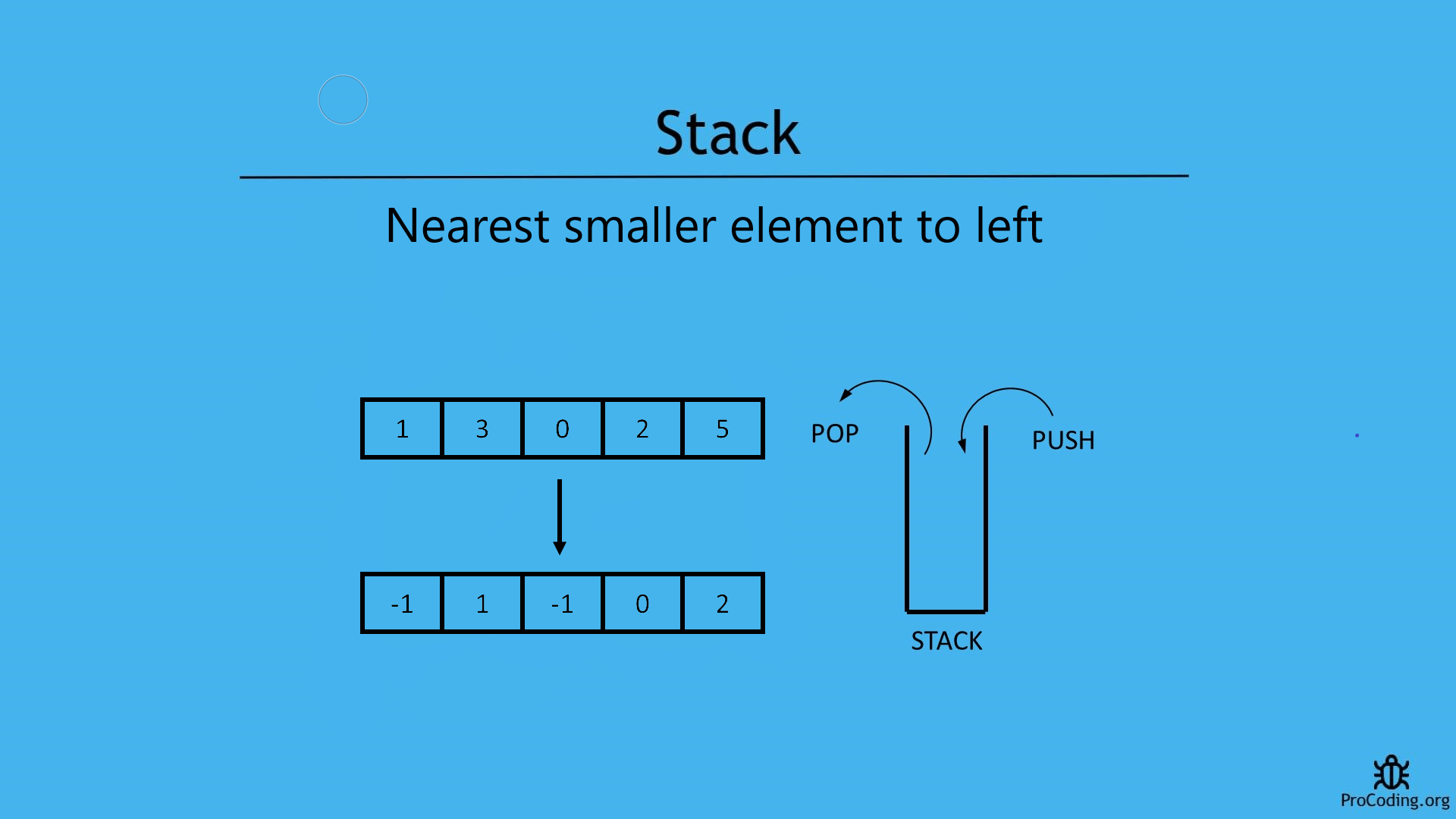#### Problem

In case of this problem, an array is given and we have to find first smaller element to the left of current element. Similalrly, find the smaller elements for each element in the array and if greater element is not available then return a default value based on the problem.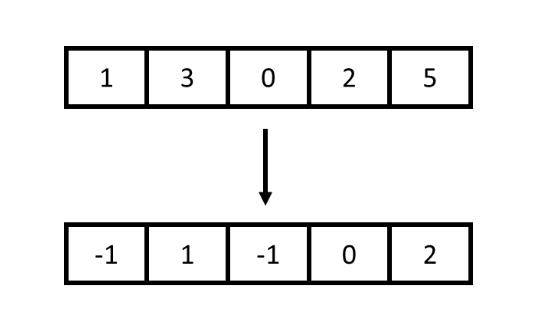##### Example -
```Input: [1, 3, 0, 2, 5]
Output: [-1, 1, -1, 0, 2]

Input: [1, 6, 4, 10, 2, 5]
Output: [-1, 1, 1, 4, 1, 2]```

Few points to consider:

1. If the array is sorted in decreasing order then next smaller element to left will always be -1.
2. For the left most element, left smaller element will always be -1.

We will see two ways to solve the problems

#### Brute-force approach (using nested loops)

In this approach we will use two loops. Outer loop will iterate over all the array items and inner loop will iterate over all the next elements of currently pointed by outer loop. Inner loop will check, if any smaller element will be found then loop will be terminated and if smaller element is not found then `-1` will be added as result.

``````def nearest_smaller_to_left(arr):
result = []
for i in range(0, len(arr)):
for j in range(i-1, -1, -1):
if arr[i] > arr[j]:
result.append(arr[j])
break
else:
result.append(-1)

return result

arr = [6, 4, 10, 2, 5]
print(nearest_smaller_to_left(arr))``````
``````function nearestSmallerToLeft(arr) {
let result = [];
for (let i = 0; i < arr.length; i++) {
let flag = false;
for (let j = i - 1; j > -1; j--) {
if (arr[i] > arr[j]) {
result.push(arr[j]);
flag = true;
break;
}
}
if (!flag) {
result.push(-1);
}
}

return result;
}

arr = [6, 4, 10, 2, 5];
console.log(nearestSmallerToLeft(arr));``````

#### Output

Output

`[-1, -1, 4, -1, 2]`

Although, we have solved this problem using loops but this is not an optimized solution.
Because time complexity of this solution is O(n2).

#### Using stack

###### Algorithm
NearestSmallerToRight(A)
``````1. for i = 0 to A.length
2.   if stack is empty
4.     stack.push(A[i])
5.   else if stack is not empty
6.     while (stack is not empty AND A[i] < top of stack)
7.       stack.pop()
8.     if stack is empty
10.    else
12.    stack.push(A[i])
13. return result``````

In case of this approach, we are using a stack.

We have to start traversing elements of the given array from the start i.e., leftmost element and we will be putting the smallest element in the stack and whenever we get another smaller element then pop the stack and push the new element. Basically, we will be using a stack to keep values available to the left side and which are the smaller ones.

If the stack is empty the smaller element to left will be `-1` otherwise top of stack will be the smaller element. At the end we have to return result array.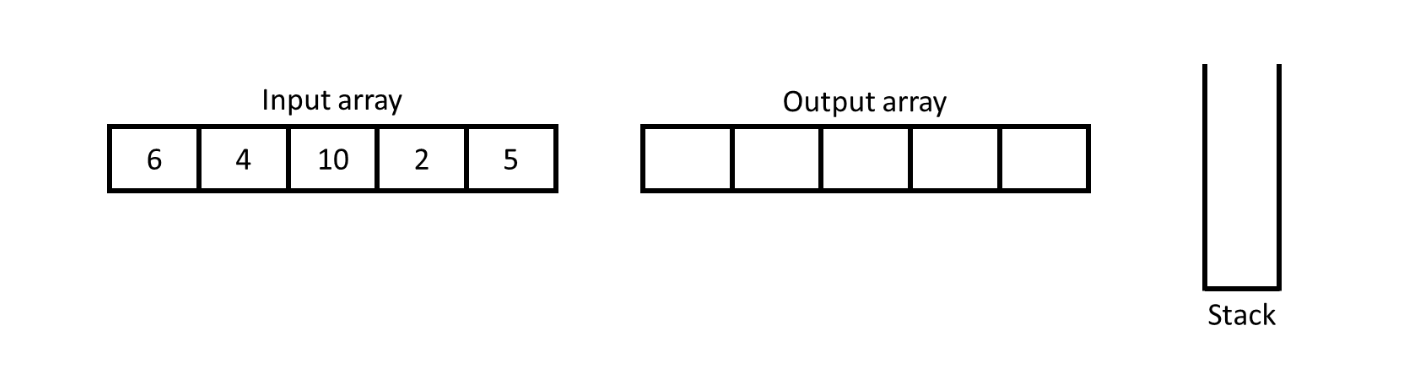Initially, input array will be given and an output array with stack will be created.Start traversing of array from the left side and for the leftmost element nearest smaller to left will be `-1` and put the value from the input array into the stack for further comparision.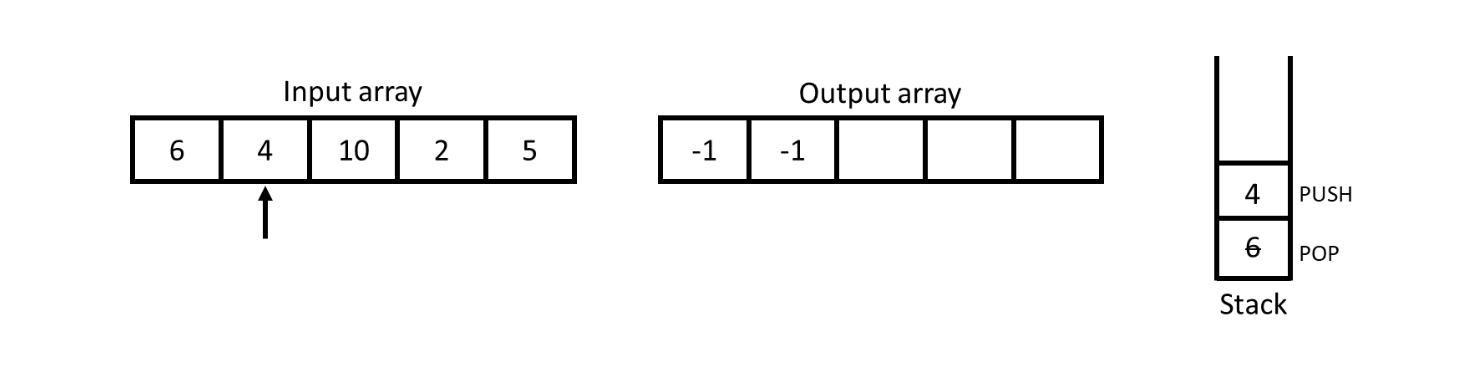For 4, stack is not empty so we have to check the top most value if it is smaller than 4 or not. Here we observe that 6 (top of stack) is not smaller than 4 so pop off 6 from stack and now stack is empty so -1 will be inserted into output array and 4 will be pushed into the stack.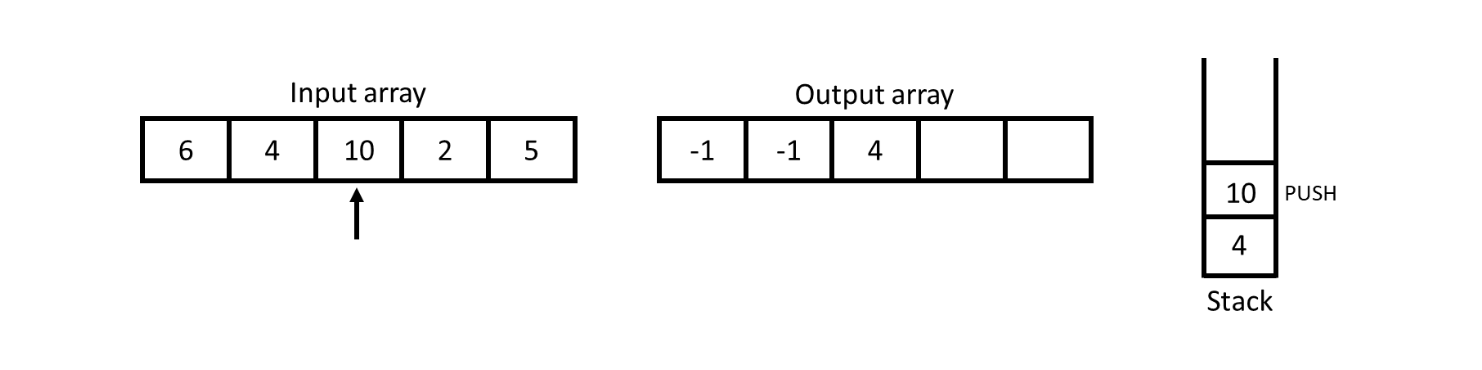For 10, stack is not empty so check the top of stack and the top of stack i.e., 4 is smaller than 10 so add 4 in the output array and push 10 into the stack.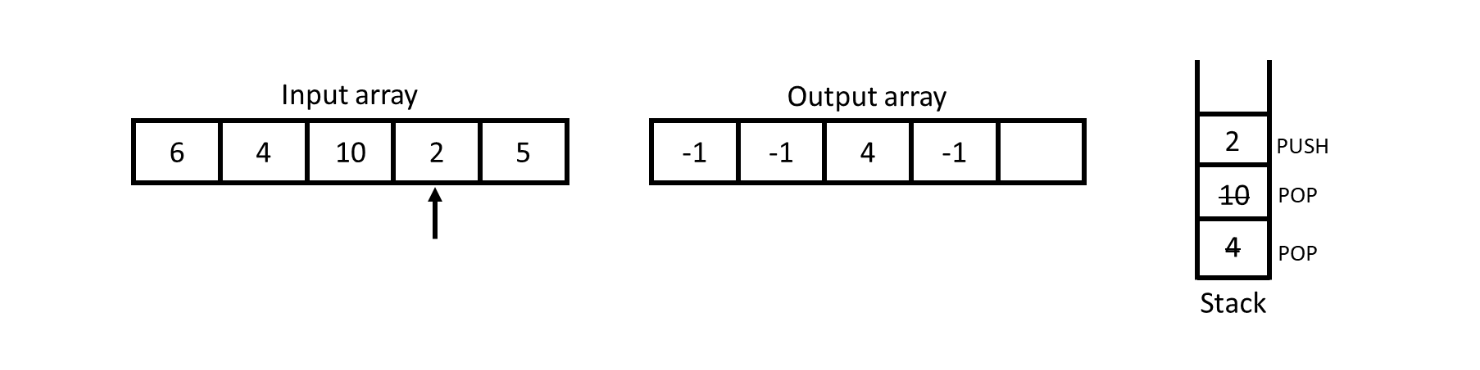For 2, the top of stack is 10 which is not smaller than 2 so pop off 10 and then the top of stack is 4 which is also not smaller than 2 so pop off 2 also. Now, stack is empty so add -1 into the output array and push 2 into the stack for further comparision.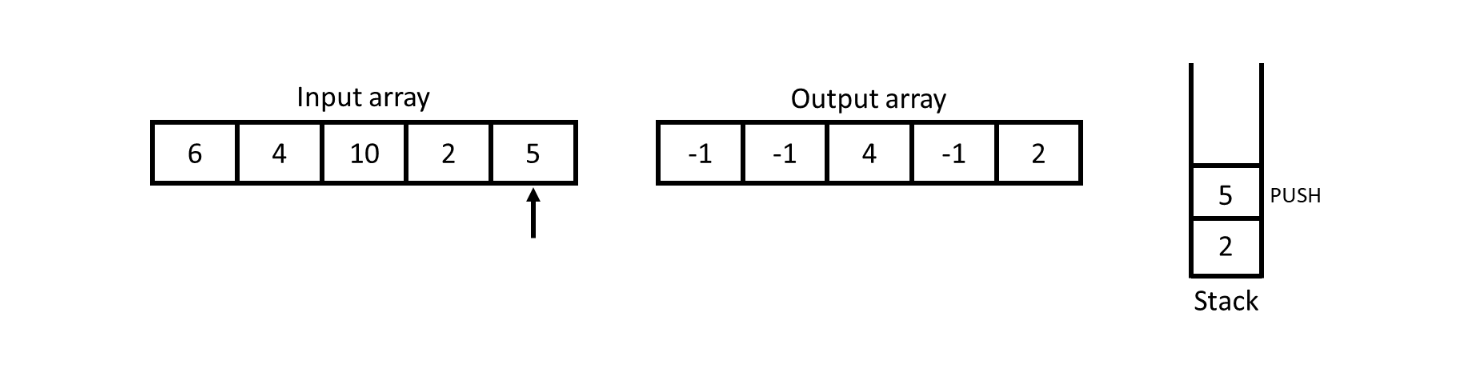For 5, the top of stack is 2 which is obviously smaller than 5 so insert 2 into the output array and push 5 into the stack.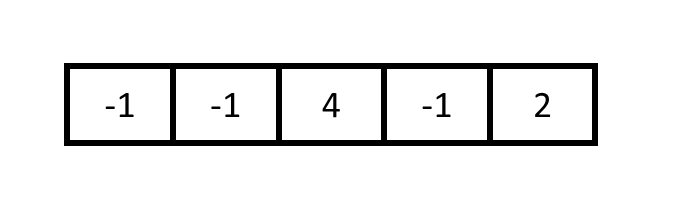At the end, return the result array to get final result.

##### Program
``````class Stack:
def __init__(self):
self.stack = []

def isEmpty(self):
return len(self.stack) == 0

def push(self, num):
self.stack.append(num)

def pop(self):
if self.isEmpty():
raise Exception('Stack Underflow')
return self.stack.pop()

def peek(self):
if self.isEmpty():
return None
return self.stack[-1]

def nearestSmallerToLeft(arr):
stack = Stack()
result = []

for i in range(0, len(arr)):
if stack.isEmpty():
result.append(-1)
stack.push(arr[i])
elif not stack.isEmpty():
while(not stack.isEmpty() and arr[i] < stack.peek()):
stack.pop()
if stack.isEmpty():
result.append(-1)
else:
result.append(stack.peek())
stack.push(arr[i])

return result

arr = [6, 4, 10, 2, 5]
print(nearestSmallerToLeft(arr))``````
``````class Stack {
constructor() {
this.stack = [];
}

isEmpty() {
return this.stack.length === 0;
}

push(num) {
this.stack.push(num);
}

pop() {
if (this.isEmpty())
throw 'Stack Underflow';
return this.stack.pop();
}

peek() {
if (this.isEmpty())
throw null;
return this.stack[this.stack.length-1]
}
}

function nearestSmallerToLeft(arr) {
const stack = new Stack();
result = [];

for (let i = 0; i < arr.length; i++) {
if (stack.isEmpty()) {
result.push(-1);
stack.push(arr[i]);
}
else if (!stack.isEmpty()) {
while (!stack.isEmpty() && arr[i] < stack.peek()) {
stack.pop();
}
if (stack.isEmpty()) {
result.push(-1);
} else {
result.push(stack.peek());
}
stack.push(arr[i]);
}
}
return result;
}

const arr = [6, 4, 10, 2, 5];
console.log(nearestSmallerToLeft(arr));``````

#### Output

Output

`[-1, -1, 4, -1, 2]`

AUTHOR### Abhishek Kumar

Software engineer | Blogger | Keen learner
Python | Django | JavaScript | React | Next.js | Express.js | C
Passionate about learning and experimenting with new things and open to more opportunities and collaborations.